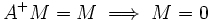Suppose$A$ is a graded ring. Let$A^+$ denote the ideal of all positively graded elements. Then, if$M$ is an$A$-graded module,$A^+M = M \implies M = 0$.